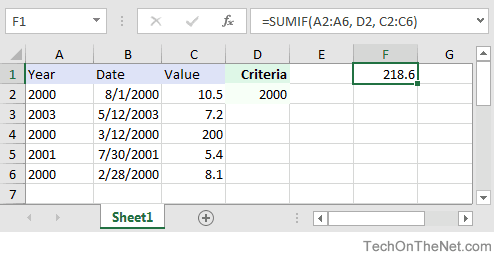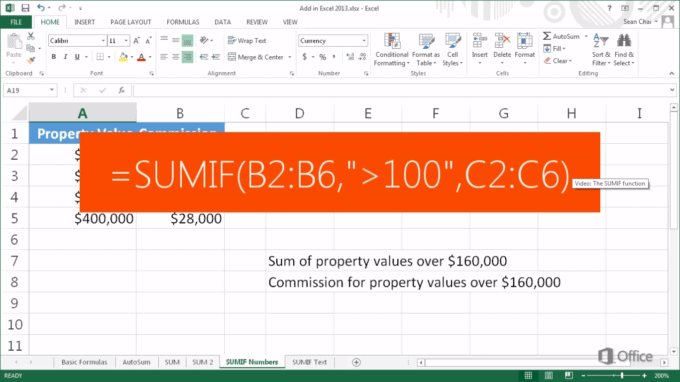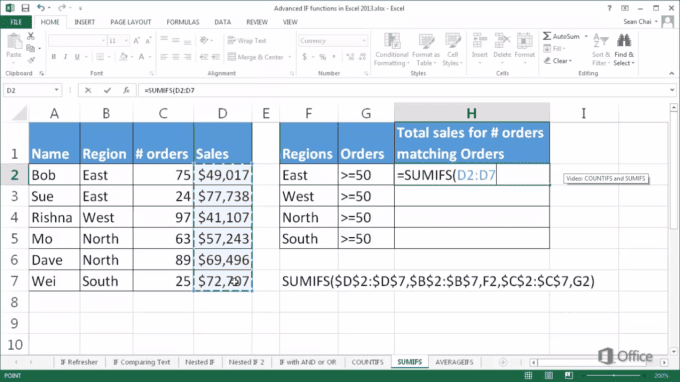# Tag Archives: criteria# How to Use the SUMIF and SUMIFS Functions in Excel

SUMIF and SUMIFS help Excel users to save time and frustration by making it easy to glean valuable information from complex datasets. You can total and analyze everything from grade values to quarterly earnings without giving yourself a massive headache.

In this tutorial, we’re going to cover:

The difference between SUMIF and SUMIFS functions.

How to use SUMIF and SUMIFS.

Common examples of formulas.

## The Basics of SUMIF Functions

Most people are familiar with Excel’s SUM function, which allows you to add together highlighted data values in a row or column. The IF function is another favorite tool that lets you see whether or not a particular data point meets a specified condition, including dates, numbers, and text.

SUMIF combines both the SUM and the IF functions to bring you a tool that not only allows you to single out information that meets essential criteria but also to find the sum of qualifying cells. You can choose a single parameter to help you isolate relevant cells, combine the data, and pull totals to gain invaluable insights into the information that you’ve collected.

### Using SUMIF Functions

SUMIF functions allow you to sort information based on one criterion, making it easy to pinpoint the data points you need. Like all Excel functions, though, you have to do a little bit of the work as well. To use SUMIF, you need to know how to express just what it is you’re looking for.

It is a worksheet function that requires a specific syntax to yield the best results. The basic syntax for the SUMIF function in Excel is:

SUMIF(range, criteria, [sum_range])

This formula may look a little complicated, especially for those just starting out with Excel, but once you get the hang of it, the function is relatively simple. There are only three different parameters that you have to keep in mind when using the SUMIF function:

Range: The cells that you wish to evaluate. It can be a column, a row, or randomly selected data points.

Criteria: The condition that must be met. Depending on your needs, you may wish to make this either text, a numeric value, or a wildcard value. It can even be another function. Just remember that all text or criteria including math symbols need to be enclosed in double quotation marks (“).

Sum_Range: This parameter is optional, but it can help when dealing with particularly large data sets. It allows you to specify the range of cells you want to sum together. If you leave this element out of the SUMIF function, as many people do, the value will default to your (Range) parameter.

### Examples of SUMIF FunctionsPeople use SUMIF functions every day in business, education, and in their personal lives. Here are some common formula examples that you might find yourself running across:

Subtotalling by a descriptor such as color: =SUMIF(descriptor_range,criteria,number_range)

Sum of cells that contain specific text: =SUMIF(range,”*text*”,sum_range)

The sum is “less than”: =SUMIF(range,”<1000″) The sum if “greater than”: =SUMIF(range,”>1000″)

The sum if cells are not equal to a specific value: =SUMIF(range,”<>value”,sum_range)

Subtotalling invoices by age: =SUMIF(age,criteria,amount)

Subtotalling by invoice number: =IF(COUNTIF(range,criteria)=1,SUMIF(range,criteria,sum_range,””)

Summing up by group or label: =IF(A2=A1,””,SUMIF(A:A,A2,B:B))

### Comparing SUMIF and SUMIFS

While the SUMIF formula allows you to differentiate between data based on a single criterion, SUMIFS will enable you to specify multiple parameters. This tool is a relatively recent development from Microsoft that only hit the markets in 2007, meaning that it’s only available in Excel 2007 or higher. With SUMIFS, you can evaluate up to 127 criteria at once instead of just one. The function offers a much more powerful tool to categorize and analyze data.

## When to Use SUMIFS Functions

SUMIF functions let you break down information based on a single differentiating factor, but that isn’t always enough. Sometimes you need to narrow data down further to analyze it, and that’s where SUMIFS comes in. You can use comparison operators like equals, less than, greater than, less than or equal to in relation to other values to gain practical insights into the real world. The basic syntax for any SUMIFS function is:

SUMIFS(sum_range, criteria_range1, criteria1, [criteria_range2, criteria2], …)

Unlike the SUMIF function, you’re required to input “[sum_range]” for SUMIFS. There are also other important parameters to define, including:

Sum_Range: The range of cells you wish to input and analyze. This field replaces the Range field used in SUMIF functions.

Criteria_Range1: This value is paired with Criteria1 to specify the range of cells to be searched.

Criteria 1: This defines the criteria that will be applied to cells in Criteria_range1. You can use text, numeric values, other cells, and even qualifying commands such as greater or less than. Any non-numeric criteria need to be enclosed in double quotes.

Criteria_range(#), Criteria(#): You can add up to 127 different criteria and range pairings onto the formula as needed.

### Examples of SUMIFS FunctionsAlthough SUMIFS is relatively new, it’s become common to see on both business and personal spreadsheets. SUMIFS formulas give users more precision and power when analyzing data sets. Here are some of the more common formulas that you might encounter:

Sum by week number: =SUMIFS(sum_range,weekrange,week)

The subtotal falling between two dates: =SUMIFS(amount,start_date,”>”&A1,end_date,”<“&B1) The sum of sales based on ID and time frame: =SUMIFS(amounts,dates,”>=”&TODAY()-30,ids,id)

### Conclusion

Excel is an invaluable tool when it comes to data analysis. Functions such as SUMIF and SUMIFS make it easy to break down information by allowing you to subtotal data based on essential criteria.

We hope that this tutorial has helped you further down the path towards becoming an Excel master. The next time that you have to subtotal data don’t forget to take advantage of the SUMIF and SUMIFS functions.